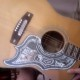### E^2(F_3) considered as a tape doughnut marked with nine (unordered) order-pairs(the “typed” image will be munged by nearly any interface,
i suppose… it looks lousy enough in mine, i know…
i never thought i’d miss DOS boxes *then*…)

^ >==========> ^
| ____________________ |
| ____________________ |
| ____________________ |
| ____________________ |
^ >==========> ^

the interior of our square
can be conveniently co-ordinatized as
I = { (x,y) | 0 < x < 1, 0 < y < 1}.
the *boundary*,$\delta(I)$, then
consists of four line segments
L = {(0,y) | 0 =< y =< 1} &
R = {(1,y) | 0 =< y =< 1}
("left" & "right"), and
T = {(x,1) | 0 =< x =< 1} &
B = {(x,0) | 0 =< x =< 1}
("top" & "bottom").
(convince yourself of this
if you can. hint: pencil
& paper.)

the Torus, T^2, can then be modeled as
"the union of I with its boundary, mod 1".

but never mind the fancy terminology.
instead we can imagine the situation
by thinking of the inside of the square
as if it were a video screen…
with the condition that when we
"move the mouse"
upwards and get to the Top (say),
we will "vanish off of" the Top
boundary and "re-emerge" at
(the corresponding point—
"having the same x-co-ordinate"—of)
the Bottom (still going upward).

& likewise for L & R
( (0, y) ~ (1, y)… i.e., for any
particular "y", we consider these
two boundary-points-of-the-square
to represent *one point* of T^2 ):
moving the mouse rightward, one
will, as it were, "go through"
the Right edge of the screen and re-
-emerge on the Left
(still going rightward).

thus far, so familiar, i hope.
using "identification diagrams" of this
kind is one of the coolest tricks
i ever learned in a math department.

now we just replace the "field of Real Numbers"
with "the" field with three elements (F_3).

all of (x,y)-space over this field
consists of *nine* ordered pairs

(and we "calculate mod 3"…
1+1+1 == 0 in this world…
but otherwise "ordinary algebra"
works…
(1, 2) + (1, 2) == (2, 4) == (2, 1),
for example…
the upshot is that we need only the
three elements of {0, 1, 2} to represent
*any integer pair* (x, y) of our
good old-fashioned "euclidean" space
):
(0,2) (1,2) (2,2)
(0,1) (1,1) (2,1)
(0,0) (1,0) (2,0).

i'll call this E^2(F_3)…
"euclidean two-space over the three-element field".

mod three arithmetic produces
"the video game effect" on this
space… one might imagine starting
with (a very flexible copy of)
(0,3) (1,3) (2,3)
(0,2) (1,2) (2,2) (3,2)
(0,1) (1,1) (2,1) (3,1)
(0,0) (1,0) (2,0) (3,0),
and then "wrapping it around"
to form a tube with each (x, 0)
covering up its corresponding (x, 3);
next (here's where "very flexible"
comes into it) "wrap around" again
the other way to produce the
(doughnut shaped) Torus by overlapping
each (3, y) with its corresponding (0, y).

now just replace the *ordered pairs of numbers*
with (unordered) pairs of "direction icons"
& draw the whole thing on the nearest
tape-doughnut to hand; voila. a pleasant

PS
ancient footnotes are everywhere:1.vlorbik

i used to routinely call the torus-
-modelled-by-identifications-on-
-the-square by the name of
“the pac-man topology”…
a chance, in fact… in front of
groups of students.

how many of them had any idea
what the devil i even meant by that
i’ll’ve had pretty much no idea…
the 80s was a long time ago it now
begins to seem… so of course
after about the turn of the century,
the rest of my remarks would be put
in terms of some more general
“video game”, more or less as
i did in the post.

still, it’ll always be the pac-man
topology to *me*. god, i hated
that game.

2.vlorbik

one frequently *gets* such chances. or used to.
consider the graph of y = tan(x), for example.

3.vlorbik

• ## (Partial) Contents Page

Vlorbik On Math Ed ('07—'09)
(a good place to start!)